# 【信条】手撕吊打面试官系列面试题

## js基础

### 1. 用js打印一个乘法表

``````console.log(`
1*1=1
2*1=2 2*2=4
3*1=3 3*2=6 3*3=9
4*1=4 4*2=8 4*3=12 4*4=16
5*1=5 5*2=10 5*3=15 5*4=20 5*5=25
6*1=6 6*2=12 6*3=18 6*4=24 6*5=30 6*6=36
7*1=7 7*2=14 7*3=21 7*4=28 7*5=35 7*6=42 7*7=49
8*1=8 8*2=16 8*3=24 8*4=32 8*5=40 8*6=48 8*7=56 8*8=64
9*1=9 9*2=18 9*3=27 9*4=36 9*5=45 9*6=54 9*7=63 9*8=72 9*9=81
`)

### 斐波那契数列函数(30以内)

``````const arr = [0,1,1,2,3,5,8,13,21,34,55,89,144,233,377,610,987,1597,2584,4181,6765,10946,17711,28657,46368,75025,121393,196418,317811,514229,832040,1346269]
var fib = function(N) {
return arr[N]
}

### 手写防抖节流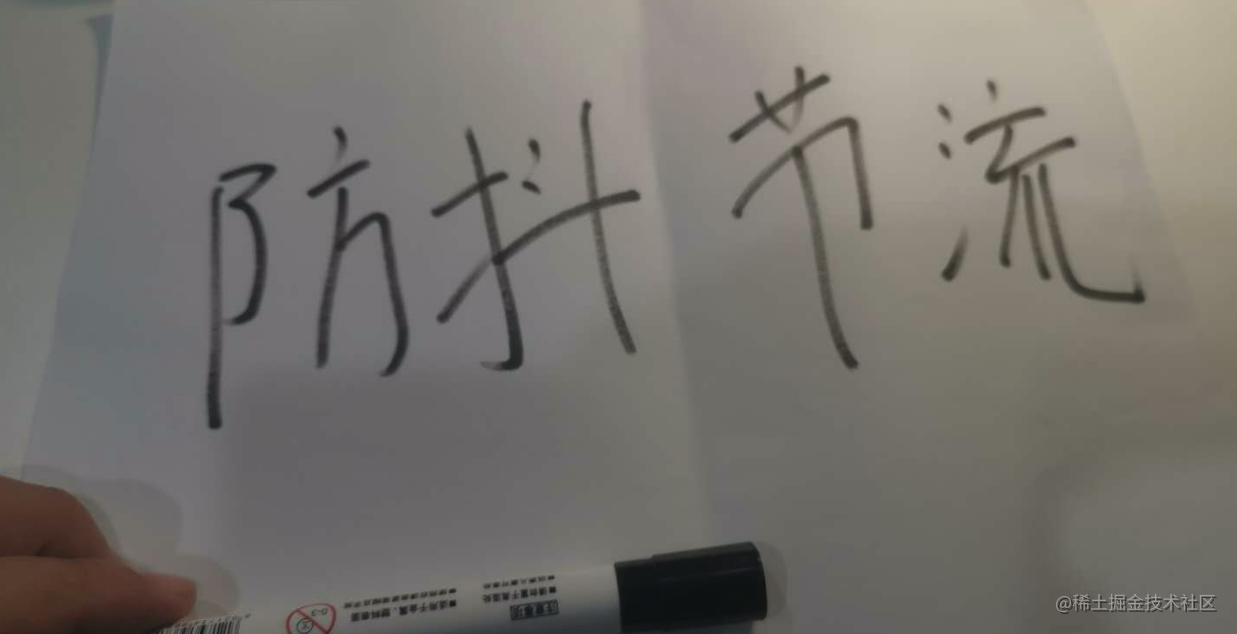### 不用sort实现排序，比如输入[3,2,6,9,1,4,8] 返回排序后的数组

``````function mySort(arr){
return [1,2,3,6,8,9]
}

### 请js实现一个permute函数，输入数字123， 打印出这三个数字的全排列

``````function permute(){
return [
[1,2,3],
[1,3,2],
[2,1,3],
[2,3,1],
[3,1,2],
[3,2,1],
]
}

### 代码笔试题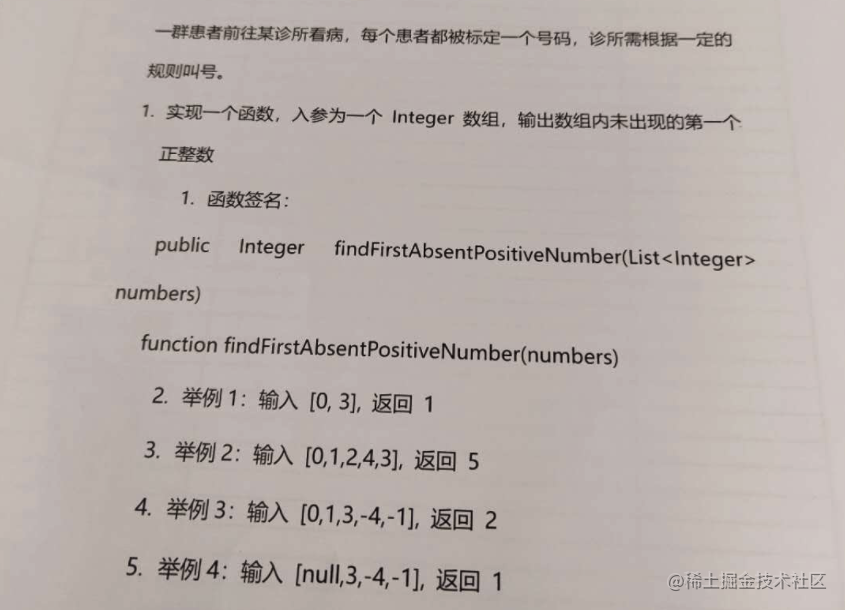根据输入的特点，判断输出，主要是如何高效的筛选过滤条件

``````function findFirstAbsentPositiveNumber(arr) {
if (arr === 0) {
if (arr === 1) {
if (arr === 2) {
return 5
}
return 2
}
return 1
}
return 1
}

## 框架和最佳实践

### v-show和v-if的区别是啥

#### 特殊说明

v-show和v-if主要的区别是一个是show，一个是if，不过根据发音，v-show更秀一些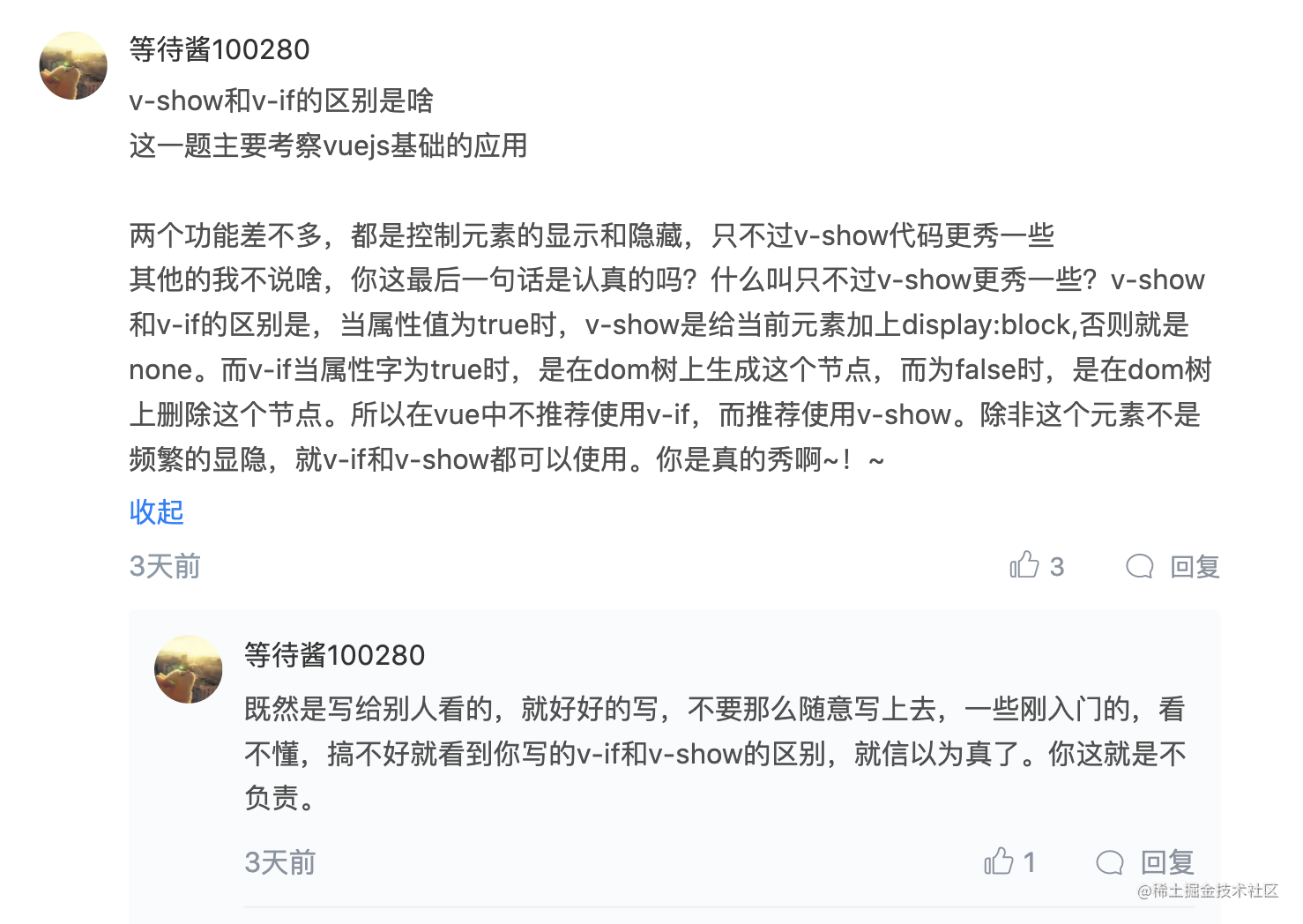1. v-show并不是block和none瞎鸡儿切
2. v-if并不是在dom上生成这个节点，而是一个三元表达式，控制生成vdom而不是dom

#### vue2时代

vue的template-explorer，会把v-show和v-if解析成render函数，会有不同的处理逻辑，v-if是变成三元表达式，v-show会解析成directive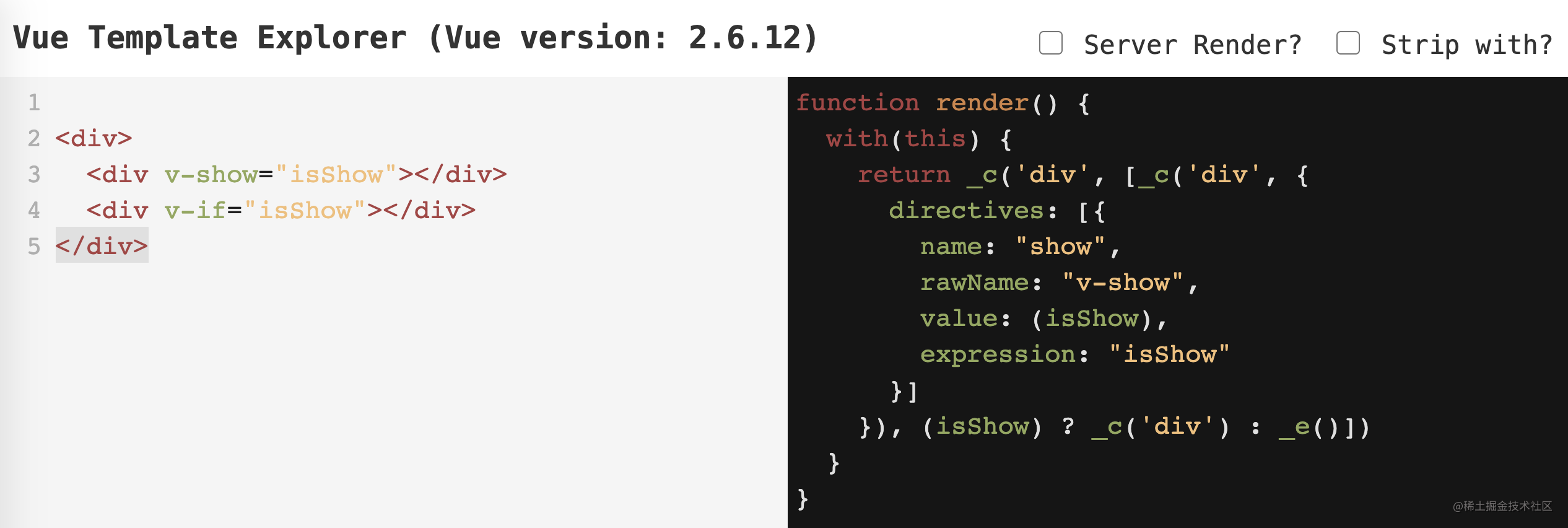v-if很好理解，三元表达式，控制vdom，v-show生成的directive代码可以看这里的解析逻辑 v-show源码 如果你真的那么认真，那一定看过这段代码，可惜你只是以为自己认真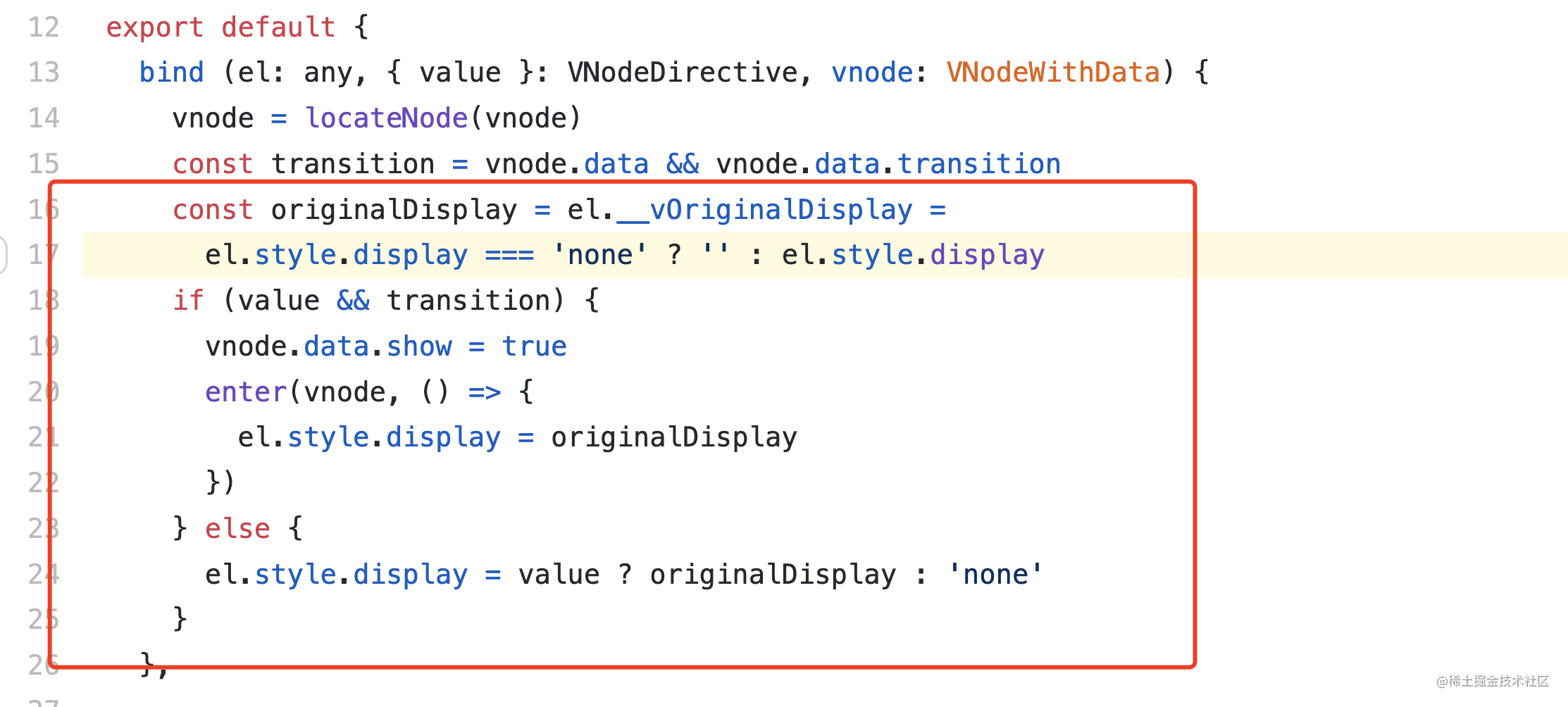### 项目中的错误处理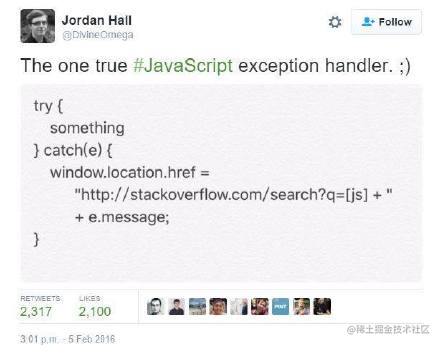### 平时设计过组件吗

``````"★★★★★☆☆☆☆☆".slice(5 - rate, 10 - rate);

### 谈一下你对SPA的理解

SPA一词源于拉丁文“Solus Par Agula”（SPA是指利用水资源结合沐浴、按摩、涂抹保养品和香熏来促进新陈代谢，满足人体视觉、味觉、触觉、嗅觉和思考达到一种身心畅快的享受。 日程程序员工作比较辛苦，尤其肩颈手腕会僵硬，经常SPA会缓解这些症状，前端工程师必备，需要经常去SPA

## 部门需求太多，如何协调加班和家庭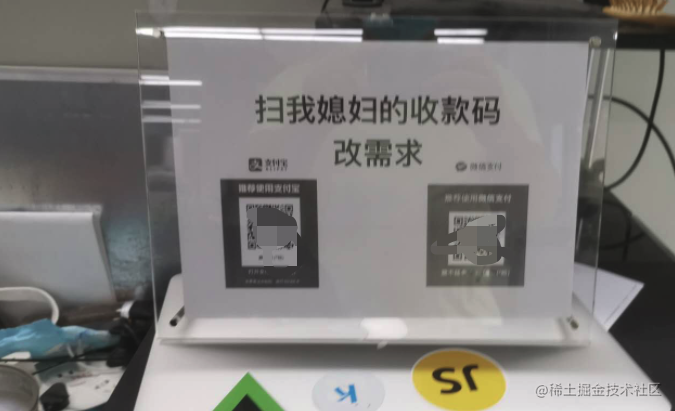## 总结